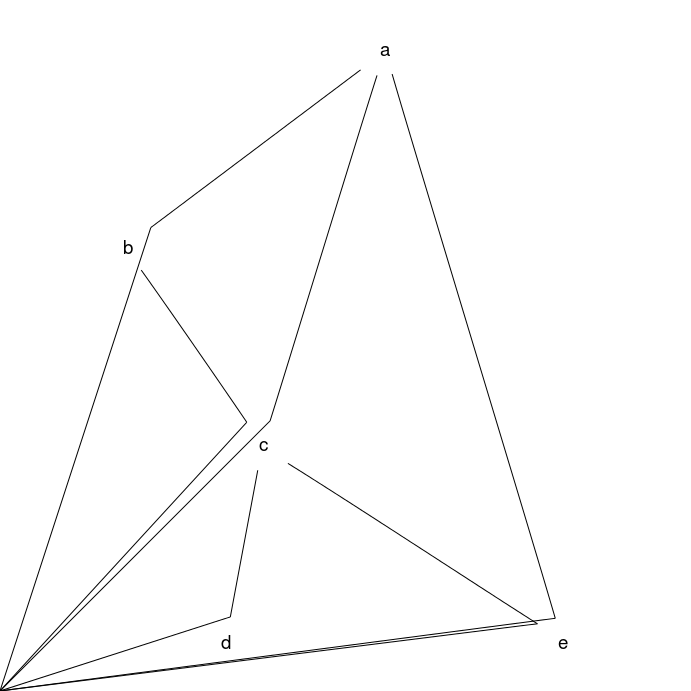# Example 1: simple undirected graph

A simple undirected graph with 5 nodes and 6 edges. The graph has been rendered with graphviz in the Linux command line, and with R packages Rgraphviz, and gridGraphviz in R.

## Graph declaration

In all cases the graph is explicitly declared with ellipse shaped nodes. The dot file edges are declared in alphabetical order as this is the (assumed) default for how Rgraphviz will pass edges internally to graphviz.

 Dot file for graphviz: `````` graph { node [shape=ellipse]; // nodes a b c d e; // edges a -- b; a -- c; a -- e; b -- c; c -- d; c -- e; } `````` R code for Rgraphviz and gridGraphviz: ```# load Rgraphviz library library(Rgraphviz) ``` ```## Loading required package: graph ## Loading required package: grid ``` ```# define nodes and edges nodes <- c("a", "b", "c", "d", "e") edgeList <- list(a=list(edges=c("b", "c", "e")), b=list(edges=c("a", "c")), c=list(edges=c("a", "b", "d", "e")), d=list(edges=c("c")), e=list(edges=c("a", "c"))) # create graph object graph <- new("graphNEL", nodes = nodes, edgeL = edgeList, edgemode = "undirected") ```

## Graph rendering:

### gridGraphviz (new)

``````>> dot -Tpng ex1.dot -o ex1.png
`````````# create Ragraph object
rag <- agopen(graph, "", attrs=list(node=list(shape="ellipse")))
```
```#plot
plot(rag)
``````library(gridGraphviz)

# create Ragraph object
rag <- agopen(graph, "", attrs=list(node=list(shape="ellipse")))
```
```#plot
grid.graph(rag)
```
```## Warning: Unsupported node shape; using 'box'
## Warning: Unsupported node shape; using 'box'
## Warning: Unsupported node shape; using 'box'
## Warning: Unsupported node shape; using 'box'
## Warning: Unsupported node shape; using 'box'
``````library(gridGraphviz)

# create Ragraph object
rag <- agopenTrue(graph, "", attrs=list(node=list(shape="ellipse")))
```
```#plot
grid.graph(rag)
```## Discussion

gridGraphviz (old) fails to produce ellipse shaped nodes, and produces "extra edges" which extend, in all cases, to the origin.